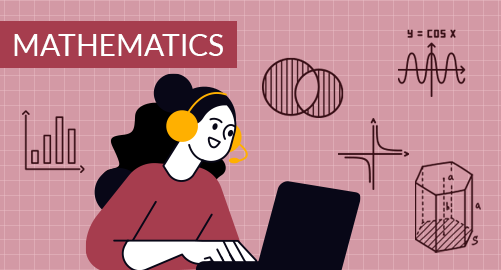#### Need Help?

Get in touch with usMathematics

Grade 7 Mathematics covers Rational Number Operations, Analyze and use proportional relationships, Analyze and solve percent problems, Generate equivalent expressions, Solve problems using equations and inequalities, Sampling and Probability, Solve Problems involving Geometry

## What you'll learn

• 1

Rational Number Operations: Relate integer and their opposites, Understand rational numbers, Add integers, Subtract integers, Add and subtract rational numbers, Multiply integers and rational numbers, Divide integers and rational numbers, Solve problems with rational numbers.

• 2

Analyze and use proportional relationships: Connect ratios, rates, and unit rates, Determine unit rates with ratios of fractions, Equivalent ratios, Constant of proportionality, Graph Proportional relationships, Apply proportional reasoning to solve problems.

• 3

Analyze and solve percent problems: Analyze precents of numbers, Connect percent and proportion, Represent and use the percent equation, Solve percent change and percent error problems, Solve markup and markdown problems, Solve simple interest problems.

• 4

Generate equivalent expressions: Write and evaluate algebraic expressions, Generate Equivalent Expressions, Simplify expressions, Expand expressions, Factor expressions, Add expressions,Subtract expressions, Analyze equivalent expressions.

• 5

Solve problems using equations and inequalities: Write two-step equations, Solve two-step equations, Solve equations using the distributive property, Solve inequalities using addition or subtraction, Solve inequalities using multiplication or division, Solve two-step inequalities, Solve multi-step inequalities.

• 6

Sampling and Probability: Population and samples, Draw inference from data, Make comparative inference about populations, Make more comparative inferences about populations, Likelihood and probability, Use probability models, Determine outcomes of compound events, probabilities of compound events, Simulate compound events.

• 7

Solve Problems involving Geometry: Solve problems involving scale drawings, Draw Geometric Figures, triangles with given conditions, Solve problems using angle relationships, circumference of a circle, area of a circle, Describe cross sections, Area of Composite Figures, Solve problems involving surface area and volume

## Skills you'll learnIdentify an appropriate mathematical rule or procedure based on the classification of a given expressionExplain how an approximated value relates to the actual valueIdentify mathematical information from graphical, numerical, analytical, and/or verbal representationsDescribe the relationships among different. representations of functions and their derivativesExplain the meaning of mathematical solutions in contextIdentify an appropriate mathematical definition, theorem, or test to applyUse precise mathematical language and appropriate units of measure and symbols